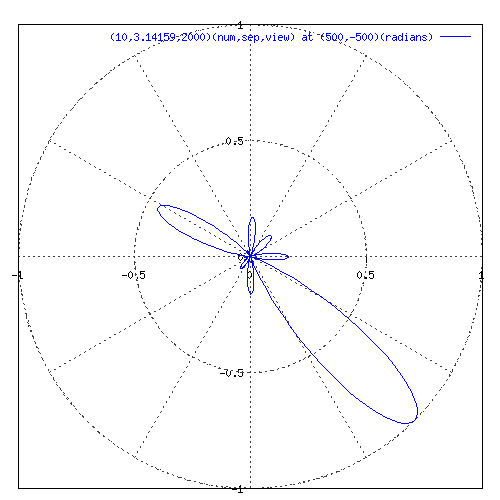# Method of Generating Graph

## Calculating power at a point

For each point the program calculates the average power over 2Pi radians of time. First it calculates the distance to the point from each antenna plus the delay added to each antenna.

phase[i]=((point[X]-ant[i][X])^2+(point[Y]-ant[i][Y])^2))^(1/2)+delay[i]

The equation for calculating power is:

Px=1/(2Pi)*integral(from 0 to 2Pi)(x(t))^2dt
x(t)=sum(from j=0 to number of antenna)cos(phase[j]+t)

This comes out nicely to:

Px=sum(from i=1 to number of antennas)(sum(from j=1 to i)(cos(phase[i]-phase[j-1])))+(number of antennas)/2

Then to keep the results comparable we normalize the results

Px/Pmax
Pmax=((number of antennas)^2/2)

these calculations are handled in the function get_point in am.c.

## Generating radiation pattern around z axis

To calculate the radiation pattern, the program finds the Power at 500 selected points around the z axis at a set radius. Then outputs the angle and power in a gnuplot compatible form. At this point the program also calculates the directivity a semi useful measure of how much power is produced in the desired direction.

Directivity=Pmax/Pvg

these calculations are handled in the function get_point in am.c.

## Setup of variables

Each antennas position is calculated by

angle=(2*M_PI)/numofant

except in the case of just one antenna which is artificially set at 0,0.
these calculations are handled in the function int_ant in am.c.

The delay from the direction point to each antenna is calculated.

these calculations are handled in the function get_phase in am.c.

gnuplot requires header information to generate the plot of the radiation pattern.

sets the graph mode in a polar format

`set polar`

sets the axis mode to nomirror
`set xtics axis nomirror`
`set ytics axis nomirror`

set grid to polar
`set grid polar`

set aspect ratio to square
`set size square`

sets the output to gif format, size 500x550 pixels using colors
white for the non-transparent background
black for borders
gray for the axes
and blue, plum and dark violet for three plotting colors.
`set terminal gif size 500,500 xffffff x000000 x404040 xff0000 xffa500 x0000ff xdda0dd x9500d3`

sets the output file name
`set output '%s'`

Does the actual plotting: plot -2Pi to 2Pi,boundaries -1,1 on both axis, And inserts a title of (number of antennas,separation between antennas,viewpoint)(and the direction point)
`plot [-2*pi:2*pi] [-1:1] [-1:1] '-' title '(%d,%g,%g)(num,sep,view) at (%g,%g)(radians)' with lines 3`

these calculations are handled in the function main in am.c.

## Method of calling program

This program was designed to have its stdout directed to gnuplot. it can be called from the command line to generate a graph using command line options. of
a.out < plot number > < Number of antennas > < distance separating antennas > < view radius > < direction point X > < direction point Y >

each position will take a -1 to indicate to use the default value

plot number values:
1 - produce a series of graphs using number of antennas from 1 to 50
2 - produce a series of graphs using distance between antennas from Pi/16 to 16Pi
3 - produce a series of graphs using the view radius from 10^0 to 10^3.5
4 - produce a series of graphs that show the pattern as the direction goes round a circle
5 - produce a series of graphs that show the pattern as the direction point moves from 10^0 to 10^3.5
6 - produce a series of graphs that show the pattern as the direction point and view radius move from 10^0 to 10^3.5
default - produce one graph using specified values. Shown below.this is demonstrated in the script runall.sh.

Matthew Hewitt